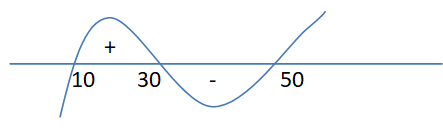Courses
Courses for Kids
Free study material
Offline Centres
MoreLast updated date: 04th Dec 2023
Total views: 384.6k
Views today: 4.84k

# A number $x$ is chosen at random from the set $\{ 1,2,3,4,...........,100\} .$ Define the event:$A =$ the chosen number $x$ satisfies $\dfrac{{(x - 10)(x - 50)}}{{(x - 30)}} \geqslant 0.$ Then $P(A)$is :Verified
384.6k+ views
Hint-Wavy curve method defined as the limit of x from the given equation. And probability is the ratio of favorable number of outcomes to the total number of outcomes. With the help of these definitions we will solve the question.

Given that:
$\Rightarrow \dfrac{{(x - 10)(x - 50)}}{{(x - 30)}} \geqslant 0$
We will use the wavy curve method to find the solution of the above equation.By wavy curve method, the solution is
$\{ 10,11,12,..........29\} U\{ 50,51,......100\}$
The value of $x = 30$ is neglected because it does not satisfy the above equation, therefore
$n(A) = 20 + 51 \\ = 71 \\$
The probability of chosen no satisfies the given equation is
$P(A) = \dfrac{{n(A)}}{{n(Givenset)}} \\ = \dfrac{{71}}{{100}} = 0.71 \\$
Hence probability of the event is 0.71

Note- This problem combines the concept of both probability and solutions of polynomial equations. For this problem the sample space is a solution of the polynomial equation which we found using a wavy curve method. In probability problems first we have to define the event and then sample space. After this we proceed further to solve the problem.